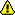You should trust both wikis because the password could be read by the particular administrators.

Clear message

# Name

cnvnp - calculate the normalized convolution function between two images using padding with zeroes and multiplication in Fourier space.

# Usage

output = cnvnp(image, ref, center=True)

## Input

image
input image (real)
ref
second input image (real) (in the alignment problems, it should be the reference image).
center
if set to True (default), the origin of the result is at the center; if set to False, the origin is at (0,0), the option is much faster, but the result is difficult to use

## Output

output
normalized convolution function between image and ref. Real. The origin of the convolution function (term ccf(0,0,0)) is located at (int[n/2], int[n/2], int[n/2]) in 3D, (int[n/2], int[n/2]) in 2D, and at int[n/2] in 1D.

# Method

• Calculation of the normalized convolution function between image f and reference image g is performed by first normalization of both images by subtracting their respective averages and by dividing them by their respective standard deviations. Next, both images are padded with zeroes to twice the size in real space, Fourier transforms of both images are calculated, their product in Fourier space calculated as `hat(f)hat(g)`, then the inverse Fourier transform, and finally the cnvnp is windowed out using the size of original images.

• In real space, this corresponds to:
• `c\nvnp(n)=(1/(nx)(sum_(k=0)^(nx-1)f((-k+n)-Ave_f)(g(k)-Ave_g)))/(sigma_f sigma_g`

• `n = -(nx)/2, ..., (nx)/2`

• with the assumption that `g(k)=0 fo\r k<0 or kgenx`

• Note: cnvnp is free from "wrap around" artifacts, although coefficients with large lag n have large error (statistical uncertainty).

# Reference

Pratt, W. K., 1992. Digital image processing. Wiley, New York.

Pawel A. Penczek

category 1
FUNDAMENTALS
category 2
FOURIER

fundamentals.py

# Maturity

stable
works for most people, has been tested; test cases/examples available.

# Bugs

None. It is perfect.